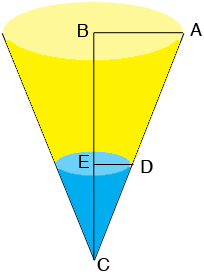SEARCH HOMEMath Central Quandaries & QueriesQuestion from Veronica, a student: A container is the shape of an inverted right circular cone has a radius of 1.00 inches at the top and a height of 5.00 inches. At the instant when the water in the container is 1.00 inches deep, the surface level is falling at the rate of -2.00 inches/second. Find the rate at which the water is being drained.Hi Veronica,

Here is my diagram of the container at a time $t$ seconds.The length of $AB$ is 1 inch and the length of $BC$ is 5 inches. Let $h(t)$ be the length of $EC$ and $r(t)$ be the length of $DE,$ both in inches. These are both functions of time.

The volume of a cone is

$V = \frac13 \pi \; r^2 h.$

Use the fact that triangles $ABC$ and $DEC$ are similar to find a relationship between $r(t)$ and $h(t)$ and then use this relationship to eliminate $r$ from the expression for the volume $V$ of the cone $DEC.$

The volume of the cone $DEC$ is a function of time and hence you can differentiate both sides of the new expression for the volume to obtain an equation with $V^\prime (t)$ on the left and an expression involving $h(t)$ and $h^\prime (t)$ on the right. Use the values of $h(t)$ and $h^\prime (t)$ when $h(t) = 1$ inch to find the value of $V^\prime (t)$ at this time.

PennyMath Central is supported by the University of Regina and The Pacific Institute for the Mathematical Sciences.